Pathways To Learning... Since 2005 Hong Kong Registered School 566985

# M - machine to mutual variation - Mathematics Dictionary

A   B   C   D   E   F   G   H   I   J   K   L   M   N   O   P   Q   R   S   T   U   V   W   X   Y   Z

machine: 1. A mechanical system that is designed to complete a task.

2. A computer algorithm considered as a complete system.

Mach number: A measure of speed of an object is a particular medium as a multiple of the speed of sound in that same medium.

Maclaurin series: A special case of a Taylor's series (see Taylors theorem) where the specific point on which the series is based has argument zero (is on the y-axis).

magic square: A square array of numbers whose sum in all rows, all columns and all (both) diagonals are the same number.

magnitude: A numerical quantity or value.

main diagonal: Also known as the leading diagonal of a matrix.

major arc: The longer of the 2 parts of circumference (arcs) defined (and split) by 2 points on the circumference of a circle.

major axis: The longer axis of an ellipse, passing through its focus.

map: Also known as a mapping.

mapping: The generalised form of a function - with arguments (inputs) and values (outputs) where an argument may have more than one value.

mass: See inertial mass.

mass centre: More commonly known as the centre of mass.

mass-energy equation: A well known equation, even amongst non-specialists, expressing energy-matter equivalence.

E=mc2

where energy E and mass m is related by c the speed of light in vacuum.

matched pairs: In experimental design, pairing units so that each member of a pair is as close as possible to the other in characteristics that might influence the outcome of a treatment.

material equivalence: A material bicondition, two sentences which share the same truth values.

material implication: The sentence of "if p then q" that is equivalent to "(not p) and q", in that they share the same truth values as sentences.

matrices: Pural of matrix.

matrix: A rectangular array of elements. For a matrix A, ai,j usually denotes the element on the ith row and jthcolumn.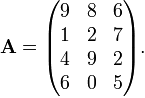so that a2,3 or a23 = 7 and a3,2 or a32 = 9

matrix of coefficients: Also known as an augmented matrix

Max: 1. A shorthand for maximum.

2. A function of 2 variables (a and b) written as Max(a,b) which always gives the larger of the 2 values.

maximal: The property of not being less than another element in a partial order. Whereas greatest is the property of being a greater (or equal) value than all other elements..

maximise: To find the parameters such that the quantity in consideration attains its greatest possible value.

maximum: The greatest in value relative to all others in consideration. The plural of the word maximum is maxima.

maximum likelihood estimation: Often abbreviated as simply MLE, it is a method to estimate the parameters of a given statistical model.

mean: A measure of central tendency of data - a location attached to a number of objects considered as one.

1. A number of measures that gives one quantity representative of the "generic" element in a set of quantities: arithmetic mean, geometric mean, harmonic mean and weighted mean.

mean absolute deviation: A measure of dispersion, it is the mean of the unsigned differences between observations and some measure of central tendency (such as mean or median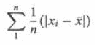mean of a g: The expectation of the random variable, also called then population mean.

mean proportional: Another class="d-title" name for the geometric mean.

mean squared error: A way of measuring the variation between the actual value and the value implied by an estimator..

mean squared deviation: Also known as mean squared error. Just as root mean square error and Root Mean Square Deviation.

mean value (of a function): The mean of a function represents the value of a constant function whose integral over the same interval equals that of the specified function.mean-value theorem: A theorem which states that given a function continuous between 2 points A=(a,f(a)) and B=(b,f(b)), there is another point "in between", C=(c,f(c)), where the gradient at c is parallel to the line through A and B.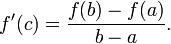measure: A concept of assigning a set of points (e.g. on a line, plane) that is a generalisation of length, area and volume etc.

measurement: The ratio of the observation of an aspect of an object to that of standard reference objects sharing in property of that same aspect.

measures of dispersion: A statistic which has certain (possibly loosely defined) properties and obeys certain (also possibly loosely defined) rules which is generally considered to be essential such that the value of from a given data set is representative of the variability (dispersion) of the data set.

The fact that these properties and rules may be loosely defined is what gives rise to different measures of dispersion: e.g. variance, standard deviation, range, interquartile range etc.

measures of location:A statistic which has certain (possibly loosely defined) properties and obeys certain (also possibly loosely defined) rules which is generally considered to be essential such that the value of from a given data set is representative of the location of the data set considered as a whole.

The fact that these properties and rules may be loosely defined is what gives rise to different measures of location: e.g. (arithmetic) mean, median, mode, mid-range etc.

mechanical advantage: The ratio of the output and input force into a mechanical system.

Mechanics: The study in mathematics of displacement (and its derivatives) and force and energy of models of physical bodies.

median: 1. A statistic of a given data set which represents the central tendency of the data set. It is either a value within the data set for which half of the set (excluding a copy of the median itself) has lower values (than the median) and the other half higher, or a value not within the data set for which half of the set is lower and the other half higher, in addition to the requirement that the median is the arithmetic mean of the two values within the data set closest to the median.

2. Also known as a midline of a triangle. It is the line segment where one of its endpoints is a vertex of a triangle and the other endpoint is the midpoint of the side oppoosite (not adjacent to) to vertex.

median test: Also known as Mood's median test, it is a test for the hypothesis that two data sets have the same median. It is performed by forming a 2x2 (2 by 2) table where the rows are the data sets and the columns are the number of data points in that set which is lower/higher than the median of the combined data set, then performing the chi-squared test on this table.

mediator: A type of variable designed to aid in identifying the relationship between a dependent and an independent vairable.

meet: Another class="d-title" name for an intersection or the act of intersection (to intersect).

mega-:An SI prefix which means 1 000 000.

member: Also known as an element. The component of a set considered as a collection. Membership of elements is the only property by which a set is distinguished from another (the Axiom of Extensionality), denoed by the symbol , where x X denotes that x is a member of the set X. This symbol is almost never used the other way around so that the set is placed before the member.

mensuration: The act of measuring of length (and higher dimension equivalents: area and volume) of components geometric figures.

Mercator Series: The Taylor series expansion of the natural logarithm ln(1 + x).

x - x2/2 + x3/3 - x4/4 + ... + (-1)n-1 xn/n + ...

Mersenne numbers: Numbers of the form 2n - 1 with n being a positive integer (equivalent to the condition that a Mersenne number must be a non-zero integer).

mesh of a partition: Given an interval which is divided into a number of non-overlapping sub-intervals by finitely many points on the interval, the mesh of the partition is the length of the sub-interval of greatest length.

method of least squares: Also known as the least squares method.

method of moments: A method of estimating parameters in the population by moments of the sample.

Metre: The SI base unit of length.

metric: A function of 2 arguments, of elements of a set, generalising the notion of distance.

A function d(x,y) such that:

(1) d(x,y) = 0 if and only if x = y

(2) d(x,y) = d(y,x) for all x,y

(3) d(x,y) ≤ d(x,z) + d(z,y)

metric space: An algebraic structure with a set defined by a metric.

metric system: A system of units of measurements based on the number 10.

metric ton: 1000 kg, also known as a tonne.

micro-:An SI prefix which means one-millionth (1/1 000 000).

micron: An alternative class="d-title" name for a micrometre.

midline: Another class="d-title" name for a median.

mid-point: A point which is equidistant between 2 points or the 2 endpoint of a line segment.

mil: An Imperial unit of length that is one-thousandth (1/1000) of an inch.

mile: A Imperial unit of length which is roughly 1.61 kilometres.

Millennium Prize problems: A set of seven mathematical problems issued by the Clay Mathematical Institute each with a prize of \$1,000,000 for a solution.

(1) the Birch and Swinnerton-Dyer conjecture.

(2) the Hodge conjecture;

(3) Navier-Stokes existence and smoothness;

(4) P versus NP;

(5) the Poincare conjecture;

(6) the Riemann hypothesis;

(7) Yang-Mills existence and mass gap;

Only one of these 7 problems have been solved as of September 2011.

milli-: An SI prefix which means one-thousandth (1/1000).

million: The class="d-title" name for one thousand thousand, 106 = 1 000 000

min: 1. A shorthand for minimum.

2. A function of 2 variables (a and b) written as min(a,b) which always gives the lesser of the 2 values.

minimal: The property of an element in a partial order which is not less than any other elements. Whereas least refers to the property os an element being lesser than all other elements.

minimal surface: A surface whose mean curvature is zero.

minimise: To find the parameters such that the quantity in consideration attains its least possible value.

minimum: The least in value relative to all others in consideration. The plural of the word minimum is minima.

minor: An element of a matrix calculated by deleting the row and column of the corresponding position in the givenmatrix, and finding the determinant of the remaining matrix (of order one less than originally).

minor arc:The shorter of the 2 parts of circumference (arcs) defined (and split) by 2 points on the circumference of a circle.

minor axis: 1. The diameter of the largest circle inscribed in an ellipse for which the endpoints are on the ellipse.

2. The diameter of the largest sphere inscribed in an ellipsoid for which the endpoints are on the ellipsoid.

minor segment: The shorter of 2 segments of a circle defined (and split) by a chord of a circle.

minuend: The quantity to be subtracted from in a subtraction, whereas the quantity to take away is the subtrahend..

minus sign: The sign -, a short horizontal bar of one alphanumeral in width, placed in the middle in its vertical position, to denote the parity of a quantity being negative or the binary operation of subtraction.

minute: 1. A unit for the measure of angle that is one sixtieth (1/60) of a degree, or 60 seconds.

2. A unit of measure of time that is one sixtieth (1/60) of an hour, or 60 seconds.

minute of arc: See minute in the sense of 1.

mixed decimal:A form of representation of a number with an integer part and a part whose quantity is between 0 and 1. e.g. 3.14

mixed fraction: A form of representation of a number with an integer part and a fraction part whose quantity is between 0 and 1. e.g. 3½.

mixed strategy: A system for a game where all available actions at all stages are assigned probabilities for which they will be picked.

mixed surd: An irrational number written as an integer (but not 1 or 0) mulitple of an nth root, the sum of an integer (not 0) with an nth root, or a combination of both.

m.k.s.: Metre, kilogram and second - the system form by the units derived from them (and the units themselves). A predecessor of the SI units (which has incorporated other base units as well).

ml: millilitre, sometimes written as mL, being one thousandth (1/1000) of a litre (L)..

Mobius function: The multiplicative function μ(n) defined on all positive integers such that:

• μ(n) = (-1)n if n has no repeated factors in its prime factorisation

• μ(n) = 0 if n has repeated factors in its prime factorisation

Mobius strip: A one-sided non-orientable surface formed by connecting one of the parallel sides of a rectangle such that pairs of diagonal vertices are identified with each other and points along the side accordingly so that the two sides are identified with each other.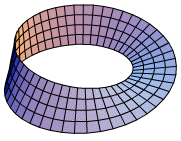modal class: The class with the ighest frequency - individual values within a class is not distinguished (even if it's possible), so that it is possible that the mode is not a value within the modal class.

mode: A statistic of a given data set which represents the central tendency of the data set. It is the value(s) which occur the most frequently within a data set. It is possible to have more than one mode.

model: A system of interlinked mathematical concept meant to represent (accurately or as an approximation) of another system.

modular arithmetic: Arithmetics with integers, based on a particular positive integer, such that two integers are considered the same (congruent) if and only if remainders from dividing by that positive integer are the same. The two numbers are said to be congruent to each other modulo n.

It can informally be thought of as the number line wrap around itself in a regular way such that the line becomes 0 again as it reaches n. Examples include: representing days of the week with Sunday as 0, Monday as 1, ... , Saturday as 6 - in this case "6+1=0" and "5+4=2" and so on; the hours in a 12-hour clock - "8+7=3" and "2-9=5" and so on.

modulo n: Modular arithmetics based around the positive integer n.

modulus: The magnitude of an object (as a non-negative real number) without regard to its directions:

1. Real Numbers - the value of the number ignoring the sign (parity)

2. Complex Numbers - A real number which can be thought of as the length of the hypotenuse formed with sides (legs) of the real and imaginary parts as perpendicular to each other

3. Algebraic expressions - The function which returns a value where the modulus is applied

4. Vectors - The magnitude of the vector

5. Matrices - The determinant of a matrix

modulus sign: A symbol consisting of 2 vertical lines, placed on either side of an expression, to indicate the modulus of a mathematical object such as number, algebraic expression, vector, matrix. e.g. |-4|, |3+4i|, |x|, |2x+3|, |5x2 + 2x + sin x|, |v|, |M| etc.

moment: 1. See moment of a force

2. The expectation of a specified integer power of the difference between a random variable and a value. For an integer power of n, the expectation of the difference between the random variable and a value v raised to the nth power is called the nth moment about v.

momenta: The plural form of the word momentum.

moment generating function: An alternative method of specifying a probability distribution. A moment generating function MX(t) of a random variable X is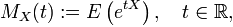Through considering the Taylor Series expansion of the function ex, the nth-order derivative of the moment generating function of a random variable evaluated at 0 is the nth moment of the random vairable.

Note that the expectation as defined above does not always exist.

moment of a force: Also known as torque.

moment of mass: Another word for the moment of inertia, it is the unwilingness of a object to rotate - dictated by the mass and the perpendicular distance between the mass and the point from which it is measured.

moment of momentum: Another word for the angular momentum of an object.

momentum: The concept of the stored (and conserved) influence of motion that can be transferred to other objects upon impact, captured by 2 modern concepts/definitions: linear momentum and angular momentum. The plural of the word momentum is momenta.

monic polynomial: A polynomial where the coefficient for the term with the highest-degree is 1.

monomial: A polynomial with only one term.

monotonic: The property of an ordered set of values where changes are all in the same direction, and may allow for no change depending on the definition used.

monotonic sequence: A non-descript decreasing sequence or increasing sequence.

mortality rate: Another class="d-title" name for death rate.

mortality tables: Another class="d-title" name for life tables.

motion: A sustained (for a period) change in position.

multinomial: An algebraic expression consisting of more than one term.

multinomial distribution: The multiple (moment and exhaustive) event analogue of the binomial distribution.

multinomial theorem: The multinomial analogue of the binomial theorem using the multinomial coefficients, which are analogues of the binomial coefficients.multiple-angle formulae: Trigonometric identities which expresses the relationship of a trigonometric function of an argument with the same trigonometric function of a multiple of the argument. e.g. double-angle formulae or triple angle formulae as shown below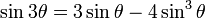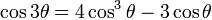multiple integration: An expression with nested integrals, also known as iterated integrals.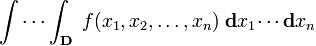multiple point: A point on a curve where the curve itersects itself. Also known as a k-tuple point where k is the number of self intersecting sections of the curve at that point.

multiple regression: Regression on a dependent variable and more than one independent variable.

multiple root: The repeated root of an equation e.g. x2+2x+1 = (x+1)(x+1). So the root -1 is repeated. If (x-a)n is a factor of a polynomial then the root a is a n-tuple root.

multiple-valued function: A mapping where an argument may correspond to more than one value.

multiplicand: The number which can be considered to be repeated added in a multiplication.

multiplication: Repeated addition and the extension of that concept to fractions, irrational, transcendental, imaginary and complex numbers; polynomials, algebraic expressions and other mathematical objects (such as matrices, vectors, vector spaces).

multiplication rule: The special case result derived from the definition of conditional probability for cases where the two events are independent.

P(A and B) = P(A) . P(B)

multiplication sign: The signs x and . which are used to denote multiplication.

multiplication table: A rectangular array of products of natural numbers which is used as an aid to children learning multiplications.

multiplicative function: 1. A function where f(xy) = f(x).f(y) for all x, y in the domain of the function, also known as a completely multiplicative function.

2. An function with positive integer arguments where f(xy)=f(x).f(y) for x,y coprime and f(1)=1.

multiplicative inverse: The number with which the specifed number multiply to give the multiplicative identity. In the case of numbers, the multiplicative identity is 1 and the inverse is also called the reciprocal (due to the way it is represented as a fraction).

multiplicity: The property of a root of a function (often a polynomial) which can be thought of as the number of roots which coincide, as seen by the factorisation of the function. The multiplicity of a root affects the appearance of the graph at the point of the root.

multiplier: The number of multiplicand to be (repeatedly) sum in a multiplication.

multivariable calculus: The study of calculus (derivatives and integrals) of functions of more than one variable. Also known as vector calculus.

multivariate data: Data of multiple variables.

multivariate distribution: A generalisation of the univariate and bivariate distributions.

mutually exclusive events: The property of 2 events whose intersection is the empty set.

mutual variation: A measure of variation of a set of paired values. e.g. covariance

A   B   C   D   E   F   G   H   I   J   K   L   M   N   O   P   Q   R   S   T   U   V   W   X   Y   Z

Genius is one percent inspiration and ninety-nine percent perspiration.